﻿ 基于有限体积法Godunov格式的管道泄漏检测模型研究 Research on Pipeline Leakage Detection Model Based on Finite Volume Method Godunov Scheme

Modeling and Simulation
Vol.07 No.03(2018), Article ID:26268,7 pages
10.12677/MOS.2018.73016

Research on Pipeline Leakage Detection Model Based on Finite Volume Method Godunov Scheme

Yue Zhao1, Ling Zhou1*, Lianwei Gao2, Yanxiu Dai2, Deyou Liu1, Yun Cao1, Tianwen Pan1

1College of Water Conservancy and Hydropower Engineering, Hohai University, Nanjing Jiangsu

2State Grid Xinyuan Company LTD Baishan Pumped Storage Power Station, Jilin Jilin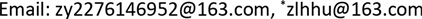Received: Jul. 9th, 2018; accepted: Jul. 26th, 2018; published: Aug. 6th, 2018ABSTRACT

Finite volume method with first order and second order Godunov schemes is presented to simulate and analyze the pipeline leakage problem. The water hammer equation is discrete by Riemann, and the MUSCL-Hancock method is used to reconstruct the two-order precision Godunov format. In order to avoid the false oscillation, the MINMOD slope limiter is introduced, and the leakage detection model is established by combining the leakage boundary conditions. The two-order Godunov scheme is consistent with the result of the characteristic line method, thus verifying the correctness of the model. The calculation analysis shows that the pressure curve of the leakage pipe is attenuated and deformed, while the pressure curve of the whole pipe does not attenuate and deform; the leakage flux affects the attenuation amplitude of the curve, and the greater the leakage flow, the greater the attenuation amplitude of the curve; the number of leakage points determines the number of inflection points at the peak curve, and the location of the leakage point determines the curve. According to the pressure curve of the leakage pipe, the leakage, the number of leakage points and the location of the leakage can be judged by the time of the inflection point of the shape and the first wave peak. This has important theoretical significance and practical guidance value for pipeline leakage monitoring.

Keywords:Finite Volume Method, Godunov Scheme, Leakage Detection

1河海大学水利水电学院，江苏 南京

2国网新源控股有限公司白山抽水蓄能电站，吉林 吉林Copyright © 2018 by authors and Hans Publishers Inc.1. 引言

2. 数学模型

2.1. 基本控制方程

$\frac{\partial u}{\partial t}+A\frac{\partial u}{\partial x}=s$ (1)

$\frac{\partial }{\partial t}{\int }_{i-1/2}^{i+1/2}udx+{f}_{i+1/2}-{f}_{i-1/2}={\int }_{i-1/2}^{i+1/2}sdx$ (2)

${f}_{i+1/2}={\overline{A}}_{i+1/2}{u}_{i+1/2}\left(t\right)=\frac{1}{2}{\overline{A}}_{i+1/2}\left\{\left(\begin{array}{cc}1& a/g\\ g/a& 1\end{array}\right){U}_{L}^{n}+\left(\begin{array}{cc}1& -a/g\\ -g/a& 1\end{array}\right){U}_{R}^{n}\right\}$ (3)

2.2. 二阶Godunov格式

${U}_{i}^{L}={U}_{i}^{n}-0.5\Delta xMINMOD\left({\sigma }_{j}^{n},{\sigma }_{j-1}^{n}\right)$ (4)

${U}_{i}^{R}={U}_{i}^{n}+0.5\Delta xMINMOD\left({\sigma }_{j}^{n},{\sigma }_{j-1}^{n}\right)$ (5)

${U}_{i}^{L*}={U}_{i}^{L}-\frac{1}{2}\frac{\Delta t}{\Delta x}\left[f\left({U}_{i}^{R}\right)-f\left({U}_{i}^{L}\right)\right]$ (6)

${U}_{i}^{R*}={U}_{i}^{R}-\frac{1}{2}\frac{\Delta t}{\Delta x}\left[f\left({U}_{i}^{R}\right)-f\left({\text{U}}_{i}^{L}\right)\right]$ (7)

${U}_{L}^{n}={U}_{i}^{R*},$ ${U}_{R}^{n}={U}_{i+1}^{L*}$ (8)

2.3. 泄漏边界条件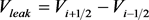(9)

${H}_{leak}={H}_{i-1/2}={H}_{i+1/2}$ (10)

${H}_{i-1/2}+\frac{a}{g}{V}_{i-1/2}={H}_{i}^{n}+\frac{a}{g}{V}_{i}^{n}-\frac{f|{V}_{i}|{V}_{i}}{2gD}\Delta x$ (11)

${H}_{i-1/2}+\frac{a}{g}{V}_{i-1/2}={H}_{i}^{n}+\frac{a}{g}{V}_{i}^{n}-\frac{f|{V}_{i}|{V}_{i}}{2gD}\Delta x$ (12)

${V}_{leak}={C}_{d}{A}_{g}\sqrt{2g\left({H}_{leak}-{H}_{out}\right)}/{A}_{1}$ (13)

3. 算例分析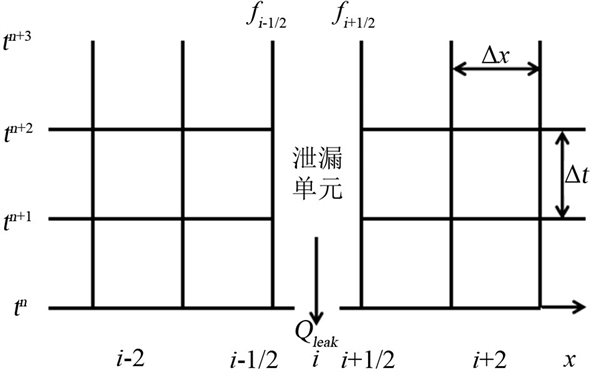Figure 1. The schematic diagram of leakage unit

3.1. 完整管道与泄漏管道对比

3.2. 泄漏参数对曲线的影响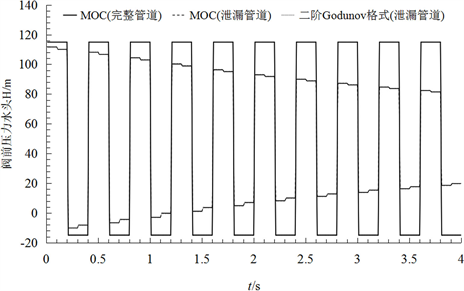(a) 方案1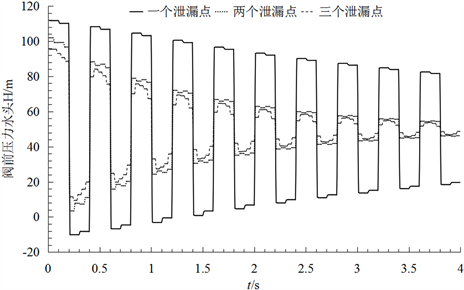(b) 方案2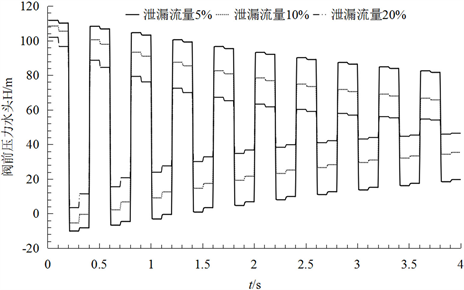(c) 方案3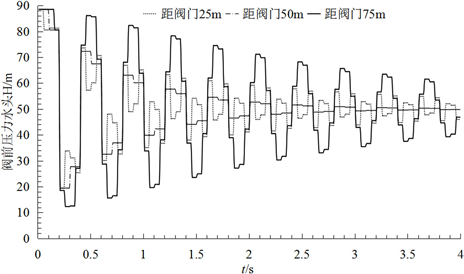(d) 方案4

Figure 2. Pressure head traces at valve

4. 结论

1) 二阶Godunov格式与MOC方法对于无摩阻的泄漏管道数值模拟结果完全一致，从而验证二阶Godunov格式模拟管道泄漏是准确可行的。

2) 管道是否发生泄漏对压力曲线的影响差别较大，管道发生泄漏会令曲线产生衰减，其中泄漏流量影响曲线的衰减幅度，即泄漏流量越大曲线衰减幅度越大。

3) 管道发生泄漏会令曲线产生变形，其中可从峰值处产生的拐点个数判断管道存在的泄漏点数量，然后从曲线向内呈现“凹”形或者向外呈现“凸”形来判断泄漏的大概位置，最后根据第一波峰发生拐点的时间确定管道发生泄漏的具体位置。

Research on Pipeline Leakage Detection Model Based on Finite Volume Method Godunov Scheme[J]. 建模与仿真, 2018, 07(03): 129-135. https://doi.org/10.12677/MOS.2018.73016

1. 1. Lee, P.J., V­Tkovsk, J.P., Simpson, A.R., et al. (2002) Leak Detection in Pipes by Frequency Response Method Using a Step Excita-tion. Journal of Hydraulic Research, 41, 221-223. https://doi.org/10.1080/00221680309499965

2. 2. Taghvaei, M., Beck, S.B.M. and Boxall, J.B. (2010) Leak Detection in Pipes Using Induced Water Hammer Pulses and Cepstrum Analysis. International Journal of Comadem, 13, 19-25.

3. 3. Krohn, N. and Busse, G. (2002) Leak Detection in Pipelines Using the Damping of Fluid Transients. Journal of Hydraulic Engineering, 128, 697-711. https://doi.org/10.1061/(ASCE)0733-9429(2002)128:7(697)

4. 4. Hamid Shamloo, A. and Candidate, A.H. (2009) Leak Detection in Pipelines by Inverse Backward Transient Analysis. Journal of Hydraulic Research, 47, 311-318. https://doi.org/10.1080/00221686.2009.9522002

5. 5. 郭新蕾, 杨开林. 管道泄漏检测的水力瞬变全频域数学模型[J]. 水利学报, 2008, 39(10): 1264-1271.

6. 6. 郭新蕾, 杨开林. 管道系统泄漏检测的瞬变水击压力波法[J]. 应用基础与工程科学学报, 2011, 19(1): 20-28.

7. 7. 郭新蕾, 杨开林, 郭永鑫. 管道泄漏检测全频域法试验验证及抗噪性研究[J]. 水利学报, 2011, 39(6): 713-720.

8. 8. 伍悦滨, 刘天顺. 基于瞬变反问题分析的给水管网漏失数值模拟[J]. 哈尔滨工业大学学报, 2005, 37(11): 1483-1485.

9. 9. 孙良, 王建林. 基于泄漏瞬变模型的管道泄漏检测与定位方法[J]. 应用基础与工程科学学报, 2012, 20(1): 159-168.

10. 10. 李若珊. 基于瞬态模拟的长输燃气管道泄漏检测方法研究[D]: [硕士学位论文]. 哈尔滨: 哈尔滨工业大学, 2005.

11. 11. 王通, 阎祥安, 李伟华. 基于谐波分析的输油管道泄漏检测机理研究[J]. 化工自动化及仪表, 2005, 32(1): 51-54.

12. 12. Zhao, M. and Ghidaoui, M.S. (2004) Godunov-Type Solutions for Water Hammer Flows. Journal of Hydraulic Engi-neering-Asce, 130, 341-348. https://doi.org/10.1061/(ASCE)0733-9429(2004)130:4(341)

13. 13. Wylie, E.B. and Suo, V.L.S. (1993) Fluid Transients. McGraw-Hill, New York, 1-40.

14. 14. Toro, E.F. (2009) Riemann Solvers and Numberical Methods for Fluid Dynamics. Verlag Berlin Heidelberg, Berlin, 115-125. https://doi.org/10.1007/b79761_4